This notebook is part of the kikuchipy documentation https://kikuchipy.org. Links to the documentation won't work from the notebook.

# Pattern matching¶

Crystal orientations can be determined from experimental EBSD patterns by matching them to a dictionary of simulated patterns of known orientations, see e.g. Chen et al. (2015), Nolze et al. (2016), Foden et al. (2019).

Here, we will demonstrate dictionary indexing (DI) using a Ni data set of 4125 EBSD patterns and a dynamically simulated master pattern from EMsoft, both of low resolution and found in the kikuchipy.data module. The pattern dictionary is generated from a discrete grid of orientations with a fixed set of projection center (PC) parameters. The true orientation is likely to fall in between multiple grid points, which means there is always a lower angular accuracy associated with each DI run. We can improve upon each orientation by letting it deviate from the discrete grid points. We do this by maximizing the similarity between experimental and simulated patterns using numerical optimization algorithms from the SciPy library. This is here called orientation refinement. We could instead keep the orientations fixed and let the PC parameters deviate from their fixed value used in the dictionary, here called projection center refinement. Finally, we can also refine both at the same time, here called orientation and projection center refinement. The need for orientation or orientation and PC refinement is discussed in e.g. Singh et al. (2017), Winkelmann et al. (2020), and Pang et al. (2020).

The term pattern matching is here used for the combined approach of DI followed by refinement.

Before we can generate a dictionary of simulated patterns, we need a master pattern containing all possible scattering vectors for a candidate phase. This can be simulated using EMsoft (Callahan and De Graef (2013) and Jackson et al. (2014)) and then read into kikuchipy.

First, we import libraries and load the small experimental Nickel test data

In :
# Exchange inline for notebook or qt5 (from pyqt) for interactive plotting
%matplotlib inline

import tempfile
import matplotlib.pyplot as plt
import hyperspy.api as hs
import numpy as np
from orix import sampling, plot, io
import kikuchipy as kp

plt.rcParams.update({"figure.facecolor": "w", "font.size": 15})

s

Out:
<EBSD, title: patterns Scan 1, dimensions: (75, 55|60, 60)>

To obtain a good match, we must increase the signal-to-noise ratio. In this pattern matching analysis, the Kikuchi bands are considered the signal, and the angle-dependent backscatter intensity, along with unwanted detector effects, are considered to be noise. See the pattern processing guide for further details.

In :
s.remove_static_background()
s.remove_dynamic_background()

Removing the static background:
[########################################] | 100% Completed |  0.3s
Removing the dynamic background:
[########################################] | 100% Completed |  1.1s

Note DI is computationally intensive and takes in general a long time to run due to all the pattern comparisons being done. To maintain an acceptable memory usage and be done within reasonable time, it is recommended to write processed patterns to an HDF5 file for quick reading during DI.
In :
#s.save("pattern_static_dynamic.h5")


## Dictionary indexing¶

### Load a master pattern¶

Next, we load a dynamically simulated Nickel master pattern generated with EMsoft, in the northern hemisphere projection of the square Lambert projection for an accelerating voltage of 20 keV

In :
energy = 20
mp = kp.data.nickel_ebsd_master_pattern_small(projection="lambert", energy=energy)
mp

Out:
<EBSDMasterPattern, title: ni_mc_mp_20kv_uint8_gzip_opts9, dimensions: (|401, 401)>
In :
mp.plot()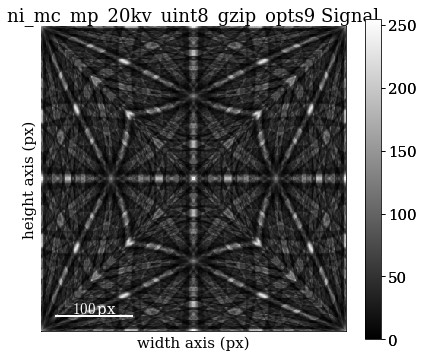The Nickel phase information, specifically the crystal symmetry, asymmetric atom positions, and crystal lattice, is conveniently stored in an orix.crystal_map.Phase

In :
ni = mp.phase
ni

Out:
<name: ni. space group: Fm-3m. point group: m-3m. proper point group: 432. color: tab:blue>
In :
ni.structure  # Element, x, y, z, site occupation

Out:
[28   0.000000 0.000000 0.000000 1.0000]
In :
ni.structure.lattice  # nm and degrees

Out:
Lattice(a=0.35236, b=0.35236, c=0.35236, alpha=90, beta=90, gamma=90)

### Sample orientation space¶

If we don't know anything about the possible crystal (unit cell) orientations in our sample, the safest thing to do is to generate a dictionary of orientations uniformly distributed in a candidate phase's orientation space. To achieve this, we sample the Rodrigues Fundamental Zone of the proper point group 432 using cubochoric sampling Singh and De Graef (2016) available in orix.sampling.get_sample_fundamental(). We can choose the average disorientation between orientations, the "resolution", of this sampling. Here, we will use a very low resolution of 4$^{\circ}$.

Note Cubochoric sampling became available in orix v0.7.
In :
rotations = sampling.get_sample_fundamental(
method="cubochoric", resolution=4, point_group=ni.point_group
)
rotations

Out:
Rotation (11935,)
[[ 0.8606 -0.3337 -0.3337 -0.1912]
[ 0.8606 -0.3397 -0.3397 -0.1687]
[ 0.8606 -0.345  -0.345  -0.1456]
...
[ 0.8606  0.345   0.345   0.1456]
[ 0.8606  0.3397  0.3397  0.1687]
[ 0.8606  0.3337  0.3337  0.1912]]

This sampling resulted in 11 935 crystal orientations. See the orix user guide on orientation sampling for further details and options for orientation sampling.

Note An average disorientation of 4$^{\circ}$ results in a course sampling of orientation space; a lower average disorientation should be used for experimental work.

### Define the detector-sample-geometry¶

Now that we have our master pattern and crystal orientations, we need to describe the EBSD detector's position with respect to the sample (interaction volume). This ensures that projecting parts of the master pattern onto our detector yields dynamically simulated patterns resembling our experimental ones. See the reference frames user guide and the EBSDDetector class for further details.

In :
signal_shape = s.axes_manager.signal_shape[::-1]
detector = kp.detectors.EBSDDetector(
shape=signal_shape,
pc=[0.421, 0.7794, 0.5049],
sample_tilt=70,
convention="tsl",
)
detector

Out:
EBSDDetector (60, 60), px_size 1 um, binning 1, tilt 0, azimuthal 0, pc (0.421, 0.221, 0.505)

Let's double check the projection/pattern center (PC) position on the detector using plot()

In :
detector.plot(coordinates="gnomonic", pattern=s.inav[0, 0].data)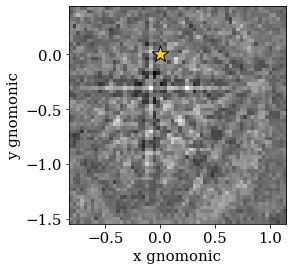### Generate dictionary¶

Now we're ready to generate our dictionary of simulated patterns by projecting parts of the master pattern onto our detector for all sampled orientations, using the get_patterns() method. The method assumes the crystal orientations are represented with respect to the EDAX TSL sample reference frame RD-TD-ND.

Note It is in general adviced to not compute the dictionary immediately, but let the dictionary indexing method handle this, by passing compute=False. This will return a LazyEBSD signal, with the dictionary patterns as a [dask array](https://docs.dask.org/en/latest/array.html).
In :
sim = mp.get_patterns(
rotations=rotations,
detector=detector,
energy=energy,
dtype_out=np.float32,
compute=True
)
sim

Creating a dictionary of (11935,) simulated patterns:
[########################################] | 100% Completed |  2.2s

Out:
<EBSD, title: , dimensions: (11935|60, 60)>

Let's inspect the three first of the 11 935 simulated patterns

In :
#sim.plot()  # Plot the patterns with a navigator for easy inspection
fig, ax = plt.subplots(ncols=3, figsize=(18, 6))
for i in range(3):
ax[i].imshow(sim.inav[i].data, cmap="gray")
ax[i].set_title(
f"($\phi_1, \Phi, \phi_2)$ = {np.array_str(euler, precision=1)}"
)
ax[i].axis("off")
fig.tight_layout()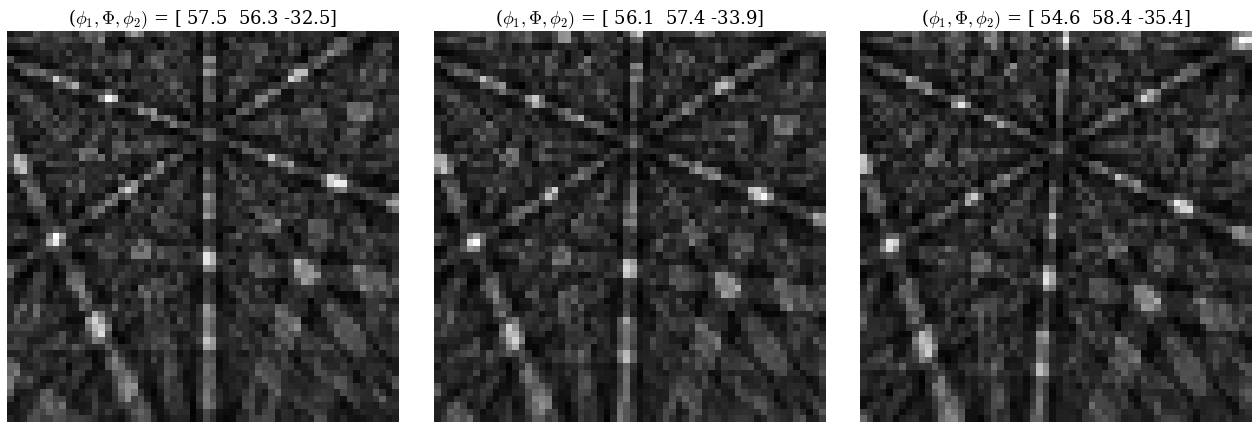### Perform indexing¶

Signal masking was added in version: 0.5.

The Kikuchi pattern signal is usually weak towards the corners of the detector, so we can pass a signal mask to only match the pixels where the mask values are False, i.e. mask out the values that are True. This convention is in line with how NumPy, Dask, scikit-image etc. defines a mask. We can pass whatever mask we want, as long as it is a boolean array of the detector shape.

In :
signal_mask = ~kp.filters.Window("circular", signal_shape).astype(bool)

p = s.inav[0, 0].data
fig, ax = plt.subplots(ncols=2, figsize=(10, 5))
ax.imshow(p * signal_mask, cmap="gray")
ax.set_title("Not used in matching")
ax.imshow(p * ~signal_mask, cmap="gray")
ax.set_title("Used in matching");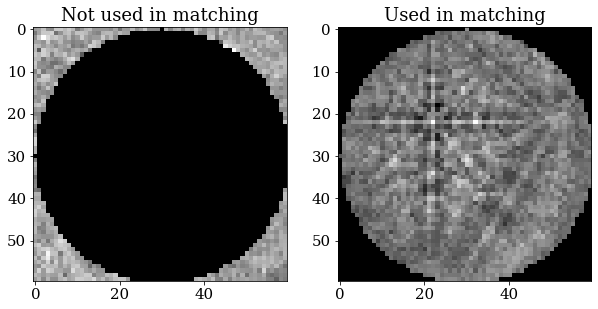Finally, let's use the dictionary_indexing() method to match the simulated patterns to our experimental patterns, using the zero-mean normalized cross correlation (NCC) coefficient $r$ Gonzalez & Woods (2017), which is the default similarity metric. Let's keep the 10 best matching orientations. A number of 4125 * 14423 comparisons is quite small, which we can do in memory all at once. However, in cases where the number of comparisons are too big for our memory to handle, we should iterate over the dictionary of simulated patterns by passing the number of patterns per iteration. To demonstrate this, we do at least 10 iterations here. The results are returned as a orix.crystal_map.CrystalMap.

In :
xmap = s.dictionary_indexing(
sim,
metric="ncc",
keep_n=10,
)
xmap

Dictionary indexing information:
Phase name: ni
Matching 4125 experimental pattern(s) to 11935 dictionary pattern(s)
NormalizedCrossCorrelationMetric: float32, greater is better, rechunk: False, signal mask: True

100%|████████████████████████████████████████████████████████████████████| 11/11 [00:05<00:00,  1.94it/s]

Out:
Phase   Orientations  Name  Space group  Point group  Proper point group     Color
0  4125 (100.0%)    ni        Fm-3m         m-3m                 432  tab:blue
Properties: scores, simulation_indices
Scan unit: px
Note *Added in version: 0.5.* Dictionary indexing of real world data sets takes a long time because the matching is computationally intensive. The [dictionary_indexing()](../reference.rst#kikuchipy.signals.EBSD.dictionary_indexing) method accepts parameters *n_per_iteration*, *rechunk* and *dtype* that lets us control this behaviour to a certain extent, so be sure to take a look at the method's docstring.

The normalized dot product can be used instead of the NCC by passing metric="ndp". A custom metric can be used instead, by creating a class which inherits from the abstract class SimilarityMetric.

The results can be exported to an HDF5 file re-readable by orix

In :
temp_dir = tempfile.mkdtemp() + "/"
xmap_file = temp_dir + "ni.h5"
io.save(xmap_file, xmap)

/home/hakon/miniconda3/envs/kp-dev/lib/python3.9/site-packages/orix/quaternion/rotation.py:312: RuntimeWarning: invalid value encountered in true_divide
np.divide(b * d - a * c, chi), np.divide(-a * b - c * d, chi)
/home/hakon/miniconda3/envs/kp-dev/lib/python3.9/site-packages/orix/quaternion/rotation.py:316: RuntimeWarning: invalid value encountered in true_divide
np.divide(a * c + b * d, chi), np.divide(c * d - a * b, chi)


### Inspect indexing results¶

So far, the orix library cannot produce any orientation color maps, pole figures etc. This has to be done in other software, like MTEX.

Here, we'll inspect the map of best matching scores and a so-called orientation similarity (OS) map, which compares the best matching orientations for each pattern to it’s nearest neighbours.

Let's get the NCC map in the correct shape from the CrystalMap’s scores property and the OS map with orientation_similarity_map() using the full list of 10 best matches per point

In :
ncc_map = xmap.scores[:, 0].reshape(*xmap.shape)
os_map = kp.indexing.orientation_similarity_map(xmap)


And plot the maps

In :
fig, ax = plt.subplots(ncols=2, figsize=(9, 3))

im0 = ax.imshow(ncc_map)
ax.axis("off")
fig.colorbar(im0, ax=ax, label="NCC")

im1 = ax.imshow(os_map)
ax.axis("off")
fig.colorbar(im1, ax=ax, label="Orientation similarity")

fig.tight_layout();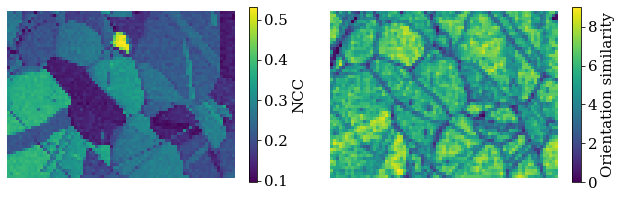We can see that some of the grains have orientations which doesn't match that well to any orientation (pattern) in the dictionary. Note that refinment will not be able to improve much upon those orientations that didn't get a good match from DI. The simplest way to improve the results for the darker grains is to reduce the orientation sampling characteristic distance. This will increase our dictionary size and thus our indexing time, but will lead to better results.

We can use the crystal map property simulation_indices to get the best matching simulated patterns from the dictionary of simulated patterns

In :
best_patterns = sim.data[xmap.simulation_indices[:, 0]].reshape(s.data.shape)
s_best = kp.signals.EBSD(best_patterns)
s_best

Out:
<EBSD, title: , dimensions: (75, 55|60, 60)>

The simplest way to visually compare the experimental and best matching simulated patterns are to plot them in the same navigator. Here, we use the highest $r$ as a navigator. When using an interactive backend like Qt5Agg, we can then move the red square around to look at the patterns in each point.

In :
ncc_navigator = hs.signals.Signal2D(xmap.get_map_data(xmap.scores[:, 0]))
hs.plot.plot_signals([s, s_best], navigator=ncc_navigator)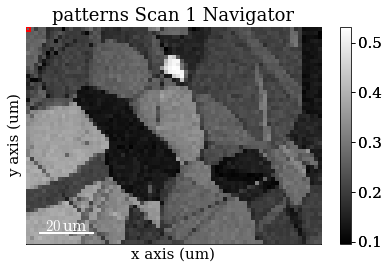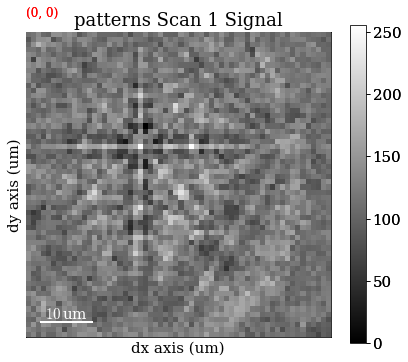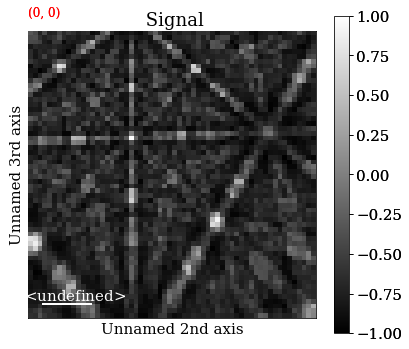Let's also plot the best matches for patterns from two grains

In :
grain1 = (0, 0)
grain2 = (30, 10)
fig, ax = plt.subplots(ncols=2, nrows=2, figsize=(10, 10))
ax[0, 0].imshow(s.inav[grain1].data, cmap="gray")
ax[0, 0].axis("off")
ax[0, 1].imshow(s_best.inav[grain1].data, cmap="gray")
ax[0, 1].axis("off")
ax[1, 0].imshow(s.inav[grain2].data, cmap="gray")
ax[1, 0].axis("off")
ax[1, 1].imshow(s_best.inav[grain2].data, cmap="gray")
ax[1, 1].axis("off")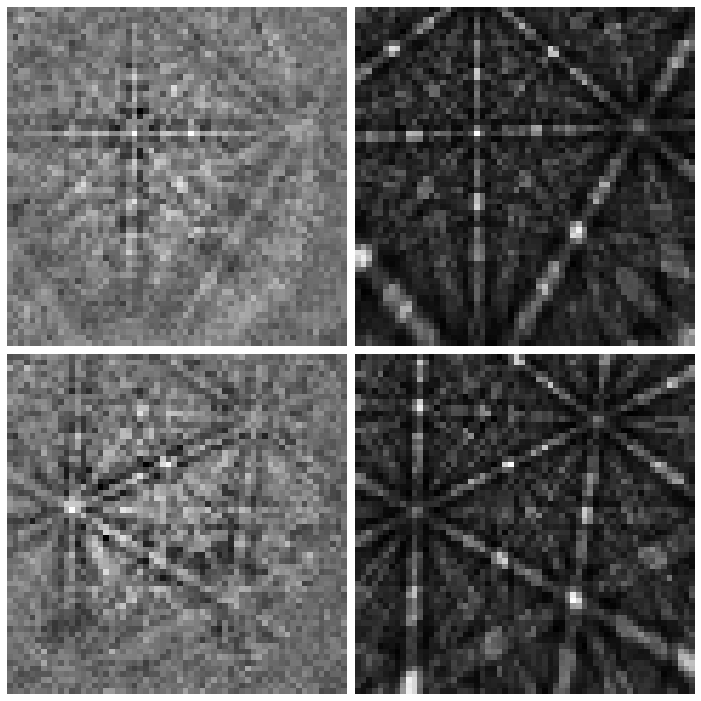## Refinement¶

Let's look at the effect of three refinement routes, all implemented as EBSD methods:

1. Refine orientations while keeping the PCs fixed: refine_orientation()
2. Refine PCs while keeping the orientations fixed: refine_projection_center()
3. Refine orientations and PCs at the same time: refine_orientation_projection_center()

For each run we will compare the maps and histograms of NCC scores before and after refinement, and also the PC parameters if appropriate.

As stated at the top, we use the numerical optimization routines from the SciPy library. For every orientation and/or PC, we want to iteratively increase the similarity (NCC score) between our experimental pattern and a new simulated pattern projected onto our EBSD detector for every iteration until the score increase from one iteration to the next is below a certain threshold, by default 0.0001 in most cases. The orientation and/or PC is updated slightly for every iteration. We have access to both local and global optimization algorithms. Consult the kikuchipy docstring methods linked below and the SciPy documentation for all available options.

Note that while we here refine orientations obtained from DI, any orientation results, e.g. from Hough indexing, can be refined with this approach, as long as an appropriate master pattern and EBSDDetector is provided, and the orientation results are passed as a CrystalMap.

### Refine orientations¶

We use refine_orientation() to refine orientations while keeping the PCs fixed, using the default local Nelder-Mead simplex method

In :
xmap_refined = s.refine_orientation(
xmap=xmap,
detector=detector,
master_pattern=mp,
energy=energy,
method="minimize",  # Default
method_kwargs=dict(method="Nelder-Mead", options=dict(fatol=1e-4)),  # Default
compute=True,
)

Refinement information:
Local optimization method: Nelder-Mead (minimize)
Keyword arguments passed to method: {'method': 'Nelder-Mead', 'options': {'fatol': 0.0001}}
Refining 4125 orientation(s):
[########################################] | 100% Completed |  1min 13.7s


Compare the NCC score maps. We use the same colorbar bounds for both maps

In :
ncc_after_orientation_refinement = xmap_refined.get_map_data("scores")

ncc_di_min = np.min(ncc_map)
ncc_di_max = np.max(ncc_map)
ncc_ori_ref_min = np.min(ncc_after_orientation_refinement)
ncc_ori_ref_max = np.max(ncc_after_orientation_refinement)

vmin = min([ncc_di_min, ncc_ori_ref_min])
vmax = max([ncc_di_max, ncc_ori_ref_max])

In :
fig, ax = plt.subplots(ncols=2, figsize=(9, 3))
im0 = ax.imshow(ncc_map, vmin=vmin, vmax=vmax)
ax.axis("off")
fig.colorbar(im0, ax=ax, label="NCC from DI")
im1 = ax.imshow(ncc_after_orientation_refinement, vmin=vmin, vmax=vmax)
ax.axis("off")
ncc_after_label = "NCC after ori. ref."
fig.colorbar(im1, ax=ax, label=ncc_after_label)
fig.tight_layout();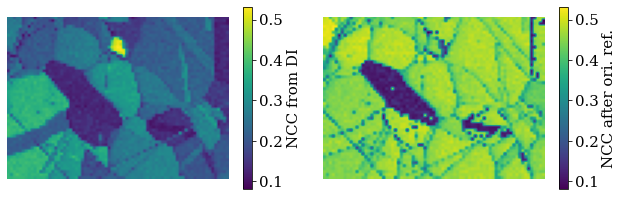Compare the histograms

In :
bins = np.linspace(vmin, vmax, 100)
fig, ax = plt.subplots(figsize=(9, 5))
_ = ax.hist(ncc_map.ravel(), bins, alpha=0.5, label="NCC from DI")
_ = ax.hist(
ncc_after_orientation_refinement.ravel(),
bins,
alpha=0.5,
label=ncc_after_label,
)
ax.set_xlabel("Normalized cross correlation (NCC) scores")
ax.set_ylabel("Frequency")
ax.legend()
fig.tight_layout();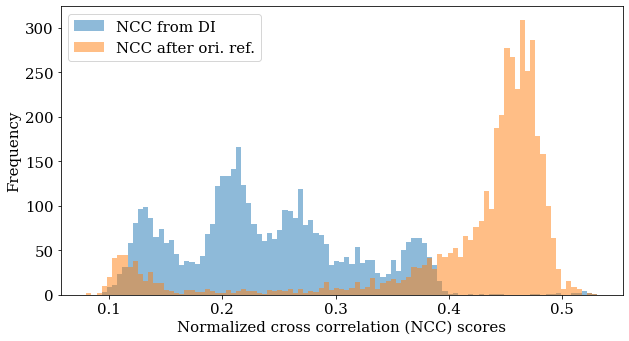We can see that orientation refinement found the best orientation (with a fixed PC) for most of the grains, but not for the ones which didn't find the correct best match in the dictionary. Clearly, the dictionary is too sparse.

### Refine projection centers¶

We use refine_projection_center() to refine PCs while keeping the orientations fixed, using the local modified Powell method. This method is also known as Bound Optimization BY Quadratic Approximation (BOBYQA), and is used in EMsoft and discussed by Singh et al. (2017). We will pass a trust_region of +/- 5% for the PC parameters to use as upper and lower bounds during refinement.

We can also pass compute=False, to do the refinement later. The results will then be a list of dask.delayed.Delayed instances. We can pass this list to kikuchipy.indexing.compute_refine_projection_center_results() and perform the refinement to retrieve the results

In :
delayed_results = s.refine_projection_center(
xmap=xmap,
detector=detector,
master_pattern=mp,
energy=energy,
method="minimize",
method_kwargs=dict(method="Powell", options=dict(ftol=1e-3)),
trust_region=[0.05, 0.05, 0.05],
compute=False,
)

Refinement information:
Local optimization method: Powell (minimize)
Keyword arguments passed to method: {'method': 'Powell', 'options': {'ftol': 0.001}}
Trust region: [0.05, 0.05, 0.05]

In :
ncc_after_pc_refinement, detector_refined = kp.indexing.compute_refine_projection_center_results(
results=delayed_results, detector=detector, xmap=xmap
)

Refining 4125 projection center(s):
[########################################] | 100% Completed |  1min 39.3s


Note that refine_orientation() and refine_orientation_projection_center() also takes the compute parameter, and there similar functions to compute the refinement results: kikuchipy.indexing.compute_refine_orientation_results() and kikuchipy.indexing.compute_refine_orientation_projection_center_results().

Let's plot the refined scores and PCs

In :
ncc_pc_ref_min = np.min(ncc_after_pc_refinement)
ncc_pc_ref_max = np.max(ncc_after_pc_refinement)

vmin2 = min([ncc_di_min, ncc_pc_ref_min])
vmax2 = max([ncc_di_max, ncc_pc_ref_max])

In :
fig, ax = plt.subplots(ncols=2, figsize=(9, 3))
im0 = ax.imshow(ncc_map, vmin=vmin2, vmax=vmax2)
ax.axis("off")
fig.colorbar(im0, ax=ax, label="NCC from DI")
im1 = ax.imshow(ncc_after_pc_refinement, vmin=vmin2, vmax=vmax2)
ax.axis("off")
ncc_after_pc_label = "NCC after PC refinement"
fig.colorbar(im1, ax=ax, label=ncc_after_pc_label)
fig.tight_layout();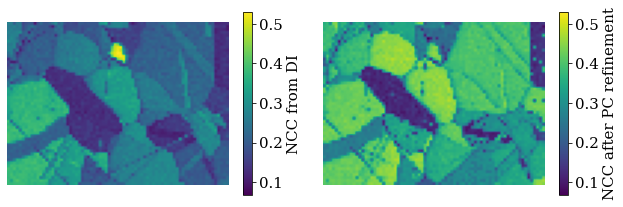Compare the histograms

In :
bins = np.linspace(vmin2, vmax2, 100)
fig, ax = plt.subplots(figsize=(9, 5))
_ = ax.hist(ncc_map.ravel(), bins, alpha=0.5, label="NCC from DI")
_ = ax.hist(
ncc_after_pc_refinement.ravel(),
bins,
alpha=0.5,
label=ncc_after_pc_label,
)
ax.set_xlabel("Normalized cross correlation (NCC) scores")
ax.set_ylabel("Frequency")
ax.legend()
fig.tight_layout();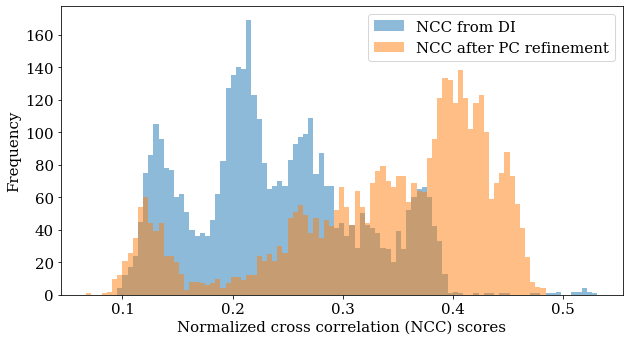In :
print(
f"PC used in DI:\t\t{detector.pc_average}\n"
f"PC after PC refinement:\t{detector_refined.pc_average}"
)

fig, ax = plt.subplots(ncols=3, figsize=(15, 3))
im0 = ax.imshow(detector_refined.pcx)
fig.colorbar(im0, ax=ax, label="Projection center x")
ax.axis("off")
im1 = ax.imshow(detector_refined.pcy)
fig.colorbar(im1, ax=ax, label="Projection center y")
ax.axis("off")
im2 = ax.imshow(detector_refined.pcz)
fig.colorbar(im2, ax=ax, label="Projection center z")
ax.axis("off")
fig.tight_layout();

PC used in DI:		[0.421 0.221 0.505]
PC after PC refinement:	[0.421 0.224 0.507]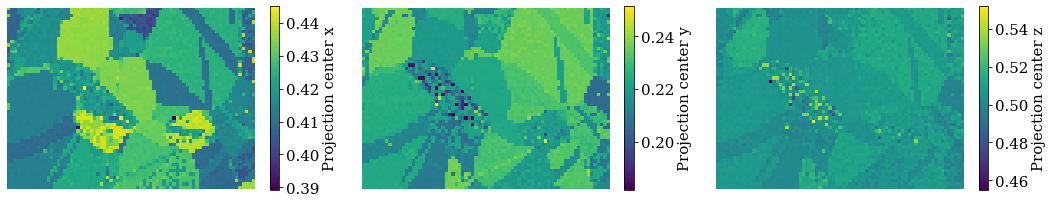### Refine orientations and projection centers¶

We use refine_orientation_projection_center() to refine orientations and PCs at the same time. For the purpose of saving time and computation resources here, we'll just do this for the upper left quarter of the data set

In :
nav_shape = s.axes_manager.navigation_shape[::-1]
y1, x1 = np.array(nav_shape) // 2
slices2d = (slice(0, y1), slice(0, x1))

In :
s2 = s.inav[slices2d[::-1]]  # HyperSpy flips the usual NumPy (row, column) to (column, row)
s2

Out:
<EBSD, title: patterns Scan 1, dimensions: (37, 27|60, 60)>
In :
xmap2 = xmap[slices2d]
xmap2

Out:
Phase  Orientations  Name  Space group  Point group  Proper point group     Color
0  999 (100.0%)    ni        Fm-3m         m-3m                 432  tab:blue
Properties: scores, simulation_indices
Scan unit: px
In :
xmap_refined2, detector_refined2 = s2.refine_orientation_projection_center(
xmap=xmap2,
detector=detector,
master_pattern=mp,
energy=energy,
method="minimize",
method_kwargs=dict(options=dict(fatol=1e-3)),
compute=True,
)

Refinement information:
Local optimization method: Nelder-Mead (minimize)
Keyword arguments passed to method: {'options': {'fatol': 0.001}, 'method': 'Nelder-Mead'}
Refining 999 orientation(s) and projection center(s):
[########################################] | 100% Completed |  1min 19.5s

In :
xmap_refined2

Out:
Phase  Orientations  Name  Space group  Point group  Proper point group     Color
0  999 (100.0%)    ni        Fm-3m         m-3m                 432  tab:blue
Properties: scores
Scan unit: px

Compare the NCC score maps. We use the same colorbar bounds for both maps

In :
ncc_after_orientation_pc_refinement = xmap_refined2.get_map_data("scores")
ncc_map2 = ncc_map[slices2d]

ncc2_di_min = np.min(ncc_map2)
ncc2_di_max = np.max(ncc_map2)
ncc_ori_pc_ref_min = np.min(ncc_after_orientation_pc_refinement)
ncc_ori_pc_ref_max = np.max(ncc_after_orientation_pc_refinement)

vmin3 = min([ncc2_di_min, ncc_ori_pc_ref_min])
vmax3 = max([ncc2_di_max, ncc_ori_pc_ref_max])

In :